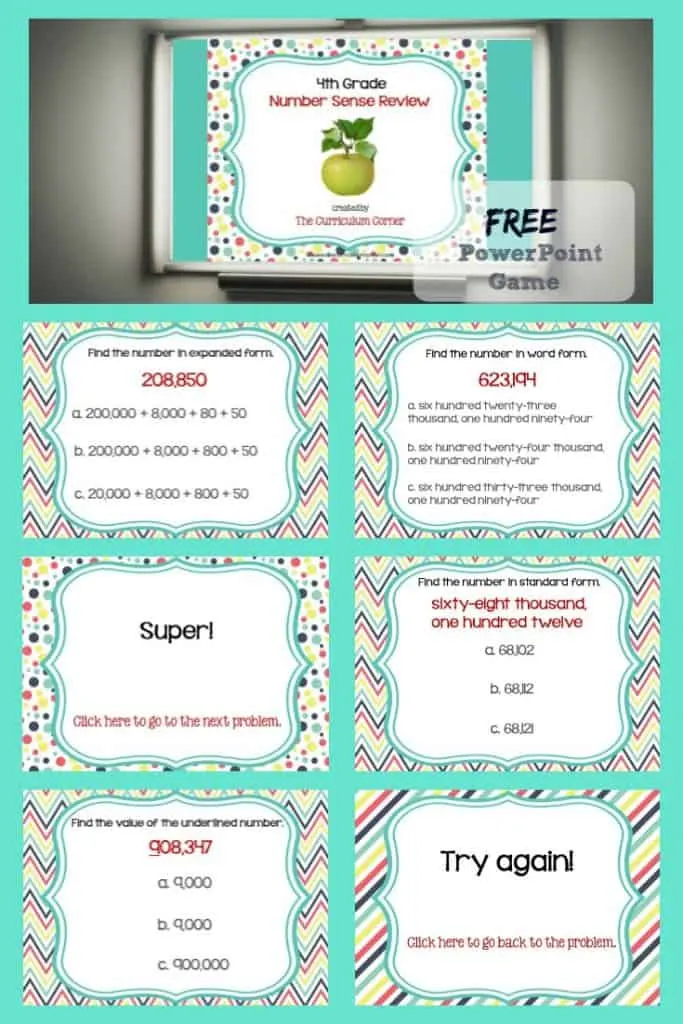# Review Game: 4th Grade Number Sense

## This 4th Grade Number Sense Review Game is designed to give your students practice with fourth grade standards throughout your unit of study.This free 4th Grade Number Sense Review Game is provided as a PowerPoint. It is designed to help you practice fourth grade standards for number sense.

This 4th Grade Number Sense Review Game is designed to match our 4th grade number sense math check.

You will find the link for the coordinating math check at the bottom of this post.

This review game was created in the hopes of it fitting into classrooms across the nation regardless of your standards.

You can use this game as review for your students before a test. Or, use it in a fifth grade classroom for a beginning of the year review.You will find problems covering each standard in this review game.

Problems for the following standards are included:

• Read and write whole numbers up to 1,000,000.
• Compare two whole numbers up to 1,000,000 using >, =, and < symbols.
• Write whole numbers as fractions. Find fractions that are equivalent to whole numbers. Name and write mixed numbers using objects or pictures. Name and write mixed numbers as improper fractions using objects or pictures.
• Use fraction models to show equivalent fractions. Find and write equivalent fractions.
• Compare two fractions with different numerators and denominators using <, =, or >.
• Use words, models, standard form and expanded form to represent decimal numbers to hundredths.
• Find all factor pairs for a whole number between 1 and 100. Determine if a whole number between 1 and 100 is a multiple of a given one-digit number.
• Use place value understanding to round multi-digit whole numbers to any given place value.

This free math game we created has been designed to touch upon each of the standards.

### Playing the Game

Correct answers throughout the game are praised with a praise slide!

To play, students need to click on the apple on the first slide and they will be taken to the first problem. When a problem is answered correctly, they will receive a slide giving them some praise. Students click on the praise and are taken to the next problem.

When students answer a problem incorrectly, they will reach some type of  “Try Again” slide and will need to click on those words to be taken back to the original problem for another attempt.

(Incorrect clicks will continue to take them to the “try again” slide.)

The game is created so that the final slide is linked to return to the first slide.